Next: How many terms to Up: Series method Previous: Series method

### Suitable point for expansion

In the above example we expanded the series around 0, and it worked. Expanding around any arbitrary point should also work, but the complexity of the resulting equations may be too high to produce a solution.

In other cases, the series may not be possible to expand at the given point. For example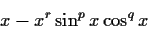cannot be expanded around x=0 because the pattern part has its leading coefficient powered to r+p, which is unknown. In such cases, most systems fail to expand, and the algorithm mentioned above will not work. Expanding around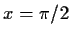does not work either, as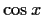will have an unknown exponent in its leading term. Expanding around x=1 (or around any other non-special value) gives a system of equations which is virtually impossible to solve.

The matching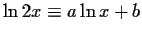, will fail if expanded at x=0 because there is no series in x (the series is in). In this case, expanding around x=1 works well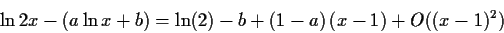giving the solution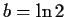, a=1 from the first two coefficients.

For simplicity, in particular for simplicity of the resulting system of equations, it is best to expand at simple values. If these do not work, expanding at a random rational should work. The main drawback of using random points of expansion is that the corresponding system of equations may be too complicated to solve.Next: How many terms to Up: Series method Previous: Series method
Gaston Gonnet
1999-07-04